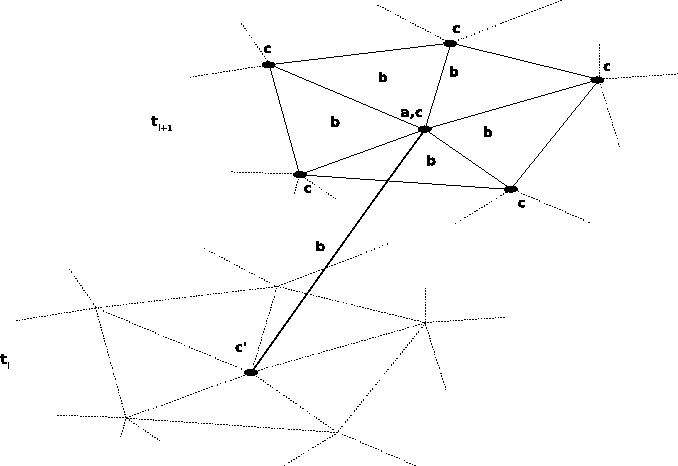# 3. 4 Finite Difference Schemes

Finite difference schemes differ from the other mentioned schemes, because it does not rely on a functional discretization, but only represents the functions used for the solution of the governing equations by a mapping of points and values. Even though this does not lead to a solution function, it can be shown that for many cases that such a method is consistent and convergent and produces adequate solutions. Especially for time-stepping, finite difference schemes are often used in combination with other discretization schemes so that the results obtained by the simulation are defined as continuous functions on single time slices.Finite difference schemes is very flexibly employable and a common basis for all operations cannot be defined by introducing a function space and treating the results of the finite difference scheme in the same manner as, e.g., the result of a finite element solution function. Finite difference schemes treat functions as defined by argument-value pairs and form interpolation functions ad-hoc for the special field of application.

The aim of this section is to find a formulation based on the systematics according to Section 2.5 and investigate the differences of the topological base operations.

Subsections
Michael 2008-01-16